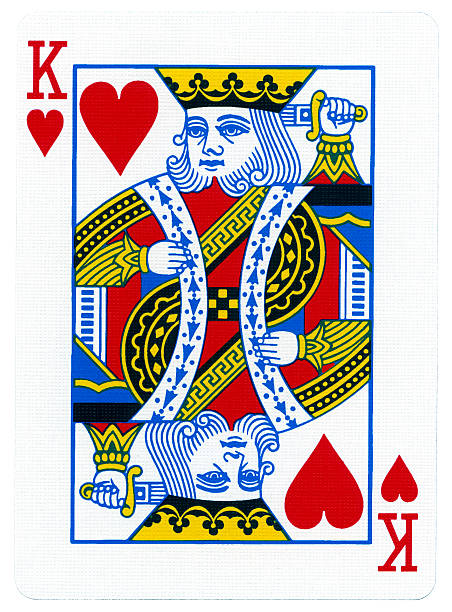## Basic Probability

Probability represents how likely an event is to occur. The probability of an outcome can be measured as $P = \frac{\#\ Outcomes}{\#\ Trials}$

To get a real measure of this, we have to observe an infinite number of trials, which is impossible.

Instead, we are stuck merely observing a sample of possible trials.

We try to make this sample as representative as we can, but sometimes logistics and chance conspire to make a sample of trials unrepresentative.

If the sample of trials is unrepresentative, then our estimate of probability may be badly wrong.

## Sampling in R

There are functions that make it very easy to sample from vectors in R.

We can use the sample() function to draw a sample of 10 individuals from this population.

Notice that when we do this twice, the samples are very rarely identical.

individuals <- 1:1000
sample(individuals, 10)
##   888 649 170 659  92 783 923 112 463   9
sample(individuals, 10, replace = TRUE)
##   220 927 485 625 928 340 329 864 200  71

## Quantifying probability

Outcome = what happens during a particular event

Sample space = the universe of possible outcomes.

Outcomes should be exhaustive in describing the sample space and the outcomes should be mutually exclusive.

When these conditions are met, then the sum of probabilities for all outcomes in the sample space is 1.

## Complex events = composites of simple events (Logical OR)sum the probabilities of simple events

e.g. probability of drawing a King from a deck of cards is the sum of the probability of drawing each of the 4 distinct kings

$1/52 + 1/52 + 1/52 + 1/52 = 4/52 = 1/13$

## Shared events = multiple simultaneous occurrences of simple events (Logical AND)

multiply the probabilities of simple events

assume that events are independent of one another

e.g. probability of drawing the Ace of spades and the 2 of clubs is the product of the probability of drawing each one separately

$1/52 * 1/52 = 1/2704$

## Set operations

The sample space can be described as a set of all possible outcomes.

For example, if we flip a coin exactly 3 times and observe the results, then the set of possible outcomes is:

$Tosses = \{(H, H, H), (H, H, T), (H, T, H), (H, T, T), (T, H, H), (T, H, T), (T, T, H), (T, T, T)\}$

## intersection (shared events)

The intersection of two sets A and B represents the elements in common to both sets.

The probability of the intersection of A and B represents the probability of the shared event A AND B. Assuming A and B are independent:

$P(A \cap B) = P(A) * P(B)$

## union (complex events)

The union of two sets A and B consists of all the shared elements of A and B.

The probability of the union of two sets represents the probability of the complex event A OR B.

To compute it we add the probabilities of A and B, and subtract the probability of the intersection between A and B, so as not to double count.

$P(A \cup B) = P(A) + P(B) - P(A \cap B)$

## Conditional Probability

Sometimes probabilities in a complex event depend on previous outcomes. To calculate the probability of A given B, we use the following formula.

$P(A|B) = \frac{P(A\cap B)}{P(B)}$

## Conditional Probabilty is fundamental

It forms the basis of frequentist statistics, as well as Bayesian statistcs, which we will talk about much more next week.